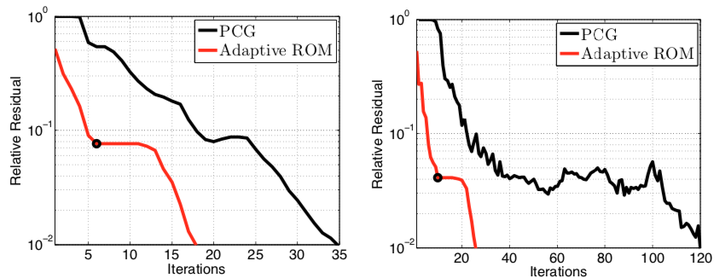# An adaptive POD-Krylov reduced-order model for structural optimizationConvergence of the relative residual for the first RHS at two online parameter instances.

### Abstract

We present an adaptive proper orthogonal decomposition (POD)-Krylov reduced-order model (ROM) for structural optimization. At each step of the optimization loop, we compute approximate solutions to the structural state and sensitivity equations using a novel POD-augmented conjugate gradient (CG) algorithm. This algorithm consists of three stages. In the first two stages, the solution component in the POD subspace is computed using a CG algorithm. Here, fast convergence is ensured due to well- conditioned reduced equations. This property results from using an energy inner product to compute the POD basis. In the third stage, the solution is refined in an adaptively-computed Krylov subspace using an augmented preconditioned CG algorithm. The dimension of the Krylov subspace is increased until the prescribed tolerance is satisfied. This methodology can be considered a reduced-order modeling technique, as it efficiently computes approximate states and sensitivities in the sum of two subspaces. The ROM is well-suited for optimization settings because its accuracy is continually improved as the optimum is approached. We report on the benchmarking of the proposed method for a direct sensitivity analysis of a parameterized V-22 tiltrotor wing panel. The results highlight the ability of the proposed method to compute approximate solutions to the structural state and sensitivity equations at a significantly lower cost than the typical augmented preconditioned CG method.

Publication
Proceedings of 8th World Congress on Structural and Multidisciplinary Optimization, Lisbon, Portugal, June 1–5, 2009